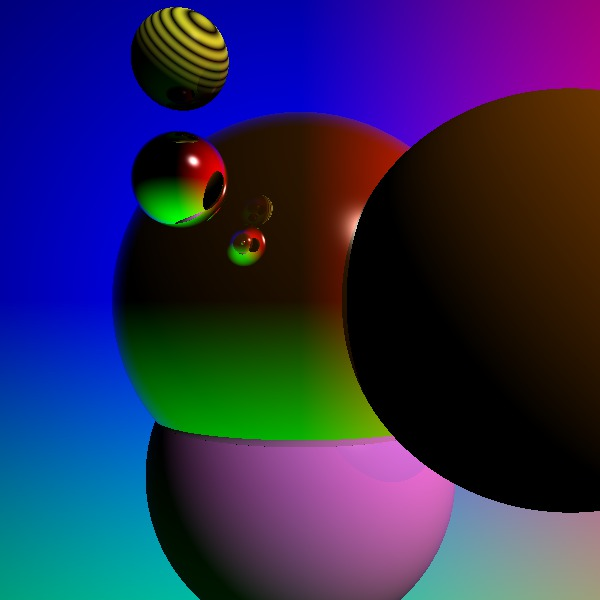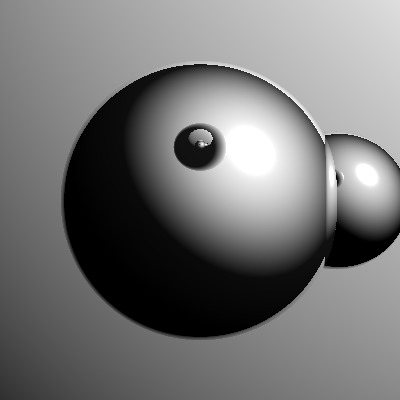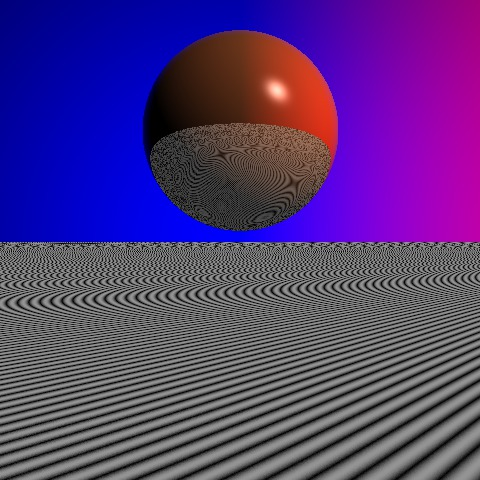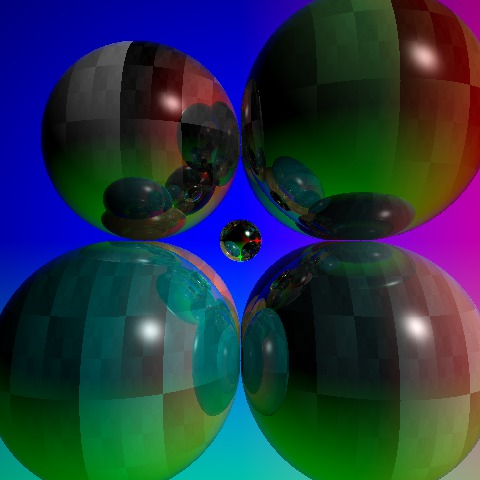# My Very First RaytracerI ran across Sebastian Sylvan's post on raytracing using signed distance functions the other day, and it occurred to me that I'd never actually written a raytracer.

So here's a simple raytracer (not using SDFs, just the usual basic approach, taking dot products and stuff). The scene description lists five spheres:

```5
x=1.2 y=0 z=3     r=.5 g=.25 b=0
x=0 y=0 z=4       r=1  g=.5  b=0  refl=0.8 rad=1.2
x=0 y=1.1 z=4     r=1  g=.5  b=1  refl=0.1 rad=1
x=-.5 y=-.5 z=2.5 r=.2 g=.2  b=.2 refl=1 (silver) rad=.2
y=-1  r=1 g=1  texture=1 refl=0.1
```

You can see that the small (rad=0.2) silver (refl=1) one is reflected in the large reflective one.

It generates this image in about a third of a second. The source is here..

Isn't it precious?

It's a tiny program, four pages of code. Some notable things it doesn't contain: matrices, transcendental functions, heap allocation, multiple kinds of geometry, or rotation. (It does invoke sqrt, floor, and pow, and use GCC's variable-sized arrays.). You could probably run it on an Arduino, especially if you rewrote it to use fixed-point math.

It does contain a tiny parser for a key-value-pair-based regular language in which objects inherit from previous objects (32 lines), a TrueColor PPM-format output file generator (12 lines), and a couple of volumetric generative textures (11 lines). Toys, but fun ones!

More images below:I was talking with Brandon Moore about writing raytracers, and I was struck by the calm beauty of the test image from his test raytracer in Haskell. This was as close as I could come with mine, because mine doesn't support changing the direction of light or the background "texture", since both are quick one-line hacks. (You could say the same of his, but they're different one-line hacks.) Worse, mine doesn't support turning off specular highlights.

```5
z=15 r=1 g=1 b=1 rad=10   A big dull sphere for camera background.
z=0  rad=40               And another for behind the camera.
z=1  rad=.33 refl=.9      A reflective sphere in front.
x=.33 rad=.17  r=0 g=0 b=0  Another to the side.
x=0 y=-.67 z=0 rad=.33  r=.5 g=.5 b=.5  And another we see only in reflection.
```I was looking at RTRT3, Mark VandeWettering's tiny realtime raytracer from 2000, and I was curious how performance compared between the two programs. So I added this code to RTRT3 and found that it was getting 6 or 7 frames per second on my netbook, compared to 96fps on his 2011 workstation:

```#include <sys/time.h>

struct timeval old_tv;

static void
fps()
{
struct timeval new_tv, diff;
double fps;
gettimeofday(&new_tv, NULL);
timersub(&new_tv, &old_tv, &diff);
old_tv = new_tv;
if (diff.tv_sec) return; /* your system is too SLOW */
fps = (1000*1000.0)/diff.tv_usec;
printf("fps: %.1lf\n", fps);
}
```

But to do an apples-to-apples comparison, I needed a scene of similar complexity: a sphere bouncing over an infinite textured plain. He also implemented shadows, which I'm still scared of. Turns out that My Very First Raytracer got about 4fps to RTRT3's 7fps, despite rendering a smaller canvas, but perhaps losing some time in generating the output file.

```2
y=-1.1  r=1 g=.5 b=.25  refl=.5
r=1 g=1 b=1  z=0 y=100000 rad=99999.7 refl=0  texture=1
```

The complex moiré aliasing in the reflected texture is totally tubular.

Mark's code makes me want to hook My Very First Raytracer up to GLUT too, in order to make it interactive.Another simple volumetric procedural texture, because checkerboards are boring.

The source code for this texture is just:

```    else if (obj->ma.texture == 'b') {
value = (((int)(point.x*128) ^ (int)(point.y*64) ^ (int)(point.z/8))
& 255) / 255.0;
}
```

and the scene is composed as follows:

```8
x=-1 y=-1  texture=b  r=1 g=1 b=1  refl=.5
y=1 b=.5
x=1 g=.5
y=-1 r=.5
z=4 g=1
y=1 b=1
x=-1 r=0
z=4.5 x=0 y=0 texture=0 refl=1 r=.2 g=.2 b=.2 rad=.2
```